### Test: ACT Science

The Ideal Gas Law is as follows: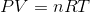is pressure as measured in Pascals,is volume as measured in cubic meters,is the number of moles of the gas,is the gas constant known as 8.314 Joules per mole times Kelvin, and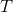is the temperature measured in Kelvin.

A class of students began studying the Ideal Gas Law and how the Pressure and the Volume relate to one another. They took 20 moles of a sample gas and kept the room at a temperature of 300 Kelvin. They then used different sized containers of the gas to limit and expand the volume. At each different volume, they measure the pressure of the gas on its container. The table they made from their results is seen in table 1.

 Temperature in Kelvin Pressure Measured in Pascals 200 Kelvin 16, 628 Pascals 400 Kelvin 33, 256 Pascals 600 Kelvin 49, 884 Pascals 800 Kelvin 66, 512 Pascals

TABLE 1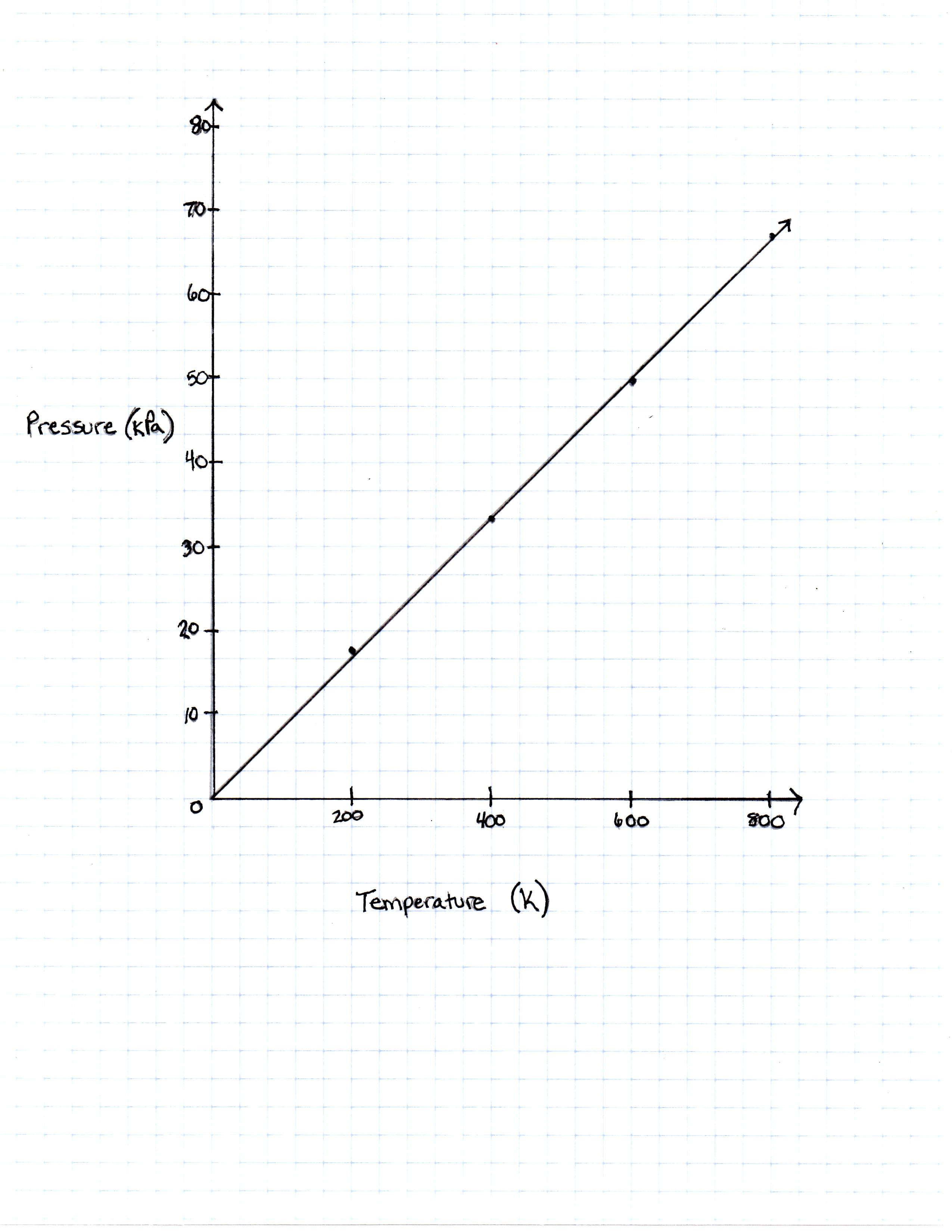FIGURE 1

The graph the students made based on the data is seen in Figure 1.

Pressure is created by the movement of the gas molecules pushing against a container. 0 Kelvin is known as absolute 0, the temperature at which all molecule movement theoretically stops.

 1 If the equation that converts temperature Kelvin to temperature in degrees Fahrenheit is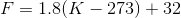what is the pressure of the sample gas at the freezing point of water, which is 32 degrees Fahrenheit?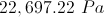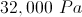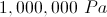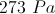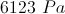1/8 questions

0%

## Access results and powerful study features!

Take 15 seconds to create an account.